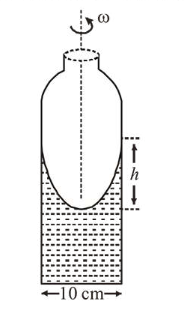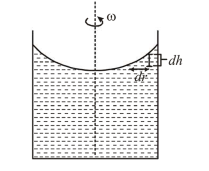# A cylindrical vessel containing a liquid is rotated about its axis so that the liquid rises at its sides as shown in the figure.

Question:

A cylindrical vessel containing a liquid is rotated about its axis so that the liquid rises at its sides as shown in the figure. The radius of vessel is $5 \mathrm{~cm}$ and the angular speed of rotation is $\omega \mathrm{rad} \mathrm{s}^{-1}$. The difference in the height, $h$ (in $\mathrm{cm}$ ) of liquid at the centre of vessel and at the side will be :1. (1) $\frac{2 \omega^{2}}{25 g}$

2. (2) $\frac{5 \omega^{2}}{2 g}$

3. (3) $\frac{25 \omega^{2}}{2 g}$

4. (4) $\frac{2 \omega^{2}}{5 g}$

Correct Option: , 3

Solution:

(3) Here, $\rho d r \omega^{2} r=\rho g d h$

$\Rightarrow \omega^{2} \int_{0}^{R} r d r=g \int_{0}^{h} d h$$\Rightarrow \frac{\omega^{2} R^{2}}{2}=g h$\        $($ Given $R=5 \mathrm{~cm})$

$\therefore h=\frac{\omega^{2} R^{2}}{2 g}=\frac{25 \omega^{2}}{2 g}$